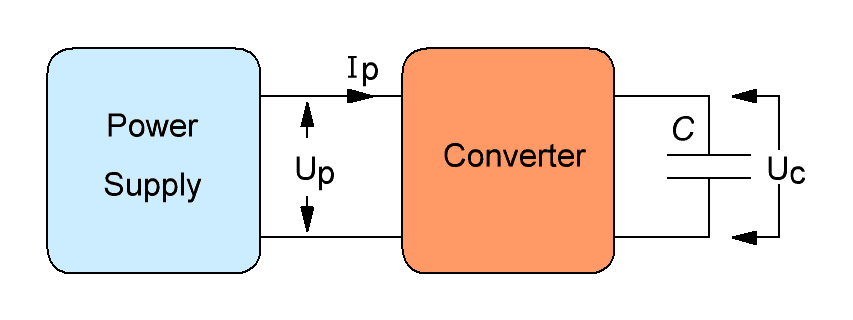Research website of Vyacheslav Gorchilin
Super efficient capacitor charging
Power supply
Voltage, V
Current, mA
-
Charging time, seconds
Input energy, mJ
Capacitor
Voltage, V
-
Capacitance, μF;
Output energy, mJ
Energy balance, E2/E1
Details
Share
Save to account
In order to share a project you have created, you need to copy the link and paste it into a blog, forum or other site:
The calculator is designed to calculate the energy balance between the Power Supply and the charged capacitor (C) through the Converter circuit. The converter is a "black box", about the structure of which we do not know anything (see figure).But we know the data of the power source: Up and Ip, and the data of the capacitor: its capacity C, and the voltage across it before and after the end of charging - Uc. The voltage before charging is usually zero volts. If the initial value is different from zero, then it is entered in the first field for the voltage across the capacitor.
The charging current gradually decreases, but it can be the same throughout the entire charge. If the current is different, then its values at the beginning and at the end of the charge are entered into two corresponding fields, and if the current is constant, then only into the second field.
Also, we know the charging time, which we also enter in the appropriate field. Based on these data, we can calculate the energy consumed by the power supply for the operation of the converter - E1, and the energy received on the capacitor after the end of charging - E2. By dividing the received and expended energies, we get their balance, which is displayed in the bottom line.
The calculation is performed according to the following method.
Saving data
This calculator can save the obtained calculations to your account. To do this, you must be registered on this site. You can save the result of the calculation, which here is called the word "project", by clicking on the button "Save to account", and then completely restore the data from the section My projects.